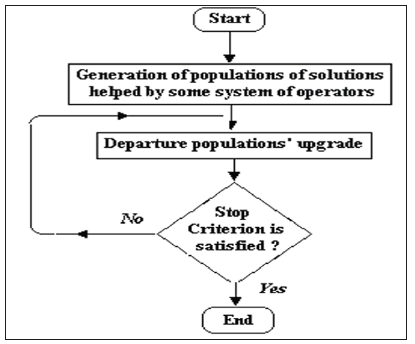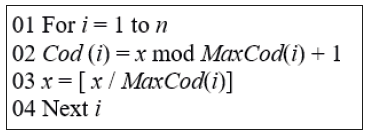• Submissions# Short Notice: The Integration of Variables Method

José Arzola-Ruiz*

Technological University of Havana, Cuba

*Corresponding author:José Arzola- Ruiz, Technological University of Havana, Cuba

Submission: June 21, 2019;Published: June 25, 2019

DOI: 10.31031/RDMS.2019.11.000756ISSN: 2576-8840
Volume11 Issue2

#### Opinion

The concept of the Integration of Variables Method is linked to the evolution of any quantity of codes helped by any set of operators to upgrade corresponding solutions populations. It general features are illustrated in Figure 1: The possible solution variants are coded in one or more variables-codes, it is generated, according to a procedure characteristic for each particular application of the method, a set of n solutions close to the optimal one. Particularly, different procedures which are characteristic for different classes of Mathematical Programming methods applied to the solution codes could be used, with selected search environments in a random, deterministic or combined way. Each particular procedure of generation and upgrading populations has to do with a concrete application variant of the method.

Figure 1:General outline of the integration of variable methods.So, the application of any heuristic derived from the Integration of Variables Method requires the definition of the following problems: a coding system for the possible solutions representation of the studied problem, a method for creating one or various initial population(s), a quality (fitness) function that allows ordering the codes according the objective function values, operators that allow the altering of the composition of solutions codes in the successive populations, stop criteria and parameters values required by the algorithm used (population’s size, probabilities associated with the application of certain operators, etc.).

Despite the general acceptance received in the last decades by other metaheuristic methods in many particular cases, difficulties have appeared in its application to optimization problems due to the following causes: there is no guarantee of obtaining the global optimal solution although generally have the tendency to do so. This tendency is frequently reduced when there is a loss of population diversity, which can lead to final populations of low quality and poor diversity, slowness of searching process in comparison with other methods, etc.

The author had developed some algorithms in the frame of the Integrations of Variables method that could in occasions overcome mentioned difficulties, particularly, increasing the diversity of the solutions populations, close to the efficient ones, that facilitate the selection of the definitive solutions by deciders as a result of being able to choose between close by the objectives functions solutions but diverse for their characteristics what has been verified in numerous application researches.

### Searching by random localization of the extreme of a function of a variable code

In Figure 2 the practical application of the Integration of variables method to the concrete case, when the searching procedure of local minima of a function of a variable code of possible solutions to the given discrete optimization task with random variation of search intervals for upgrading the successive solutions populations is illustrated.

Figure 2:Pseudocode of the localization of the extremes of a function of a variable code algorithm.In the pseudocode shown in Figure 2, in each iteration it is carried out the search of the minimum of a function of a variable code (that constitute the values, in the decimal system of numeration, of the variable-code of the looked-for solution). The initial variable code values are generated aleatorily inside the interval of possible values of the solution variable code. Searching solutions is carried out by the operator of the Localization of the minimum of the function of one independent variable method. The quality function Z could be interpreted in the same way that Genetic Algorithms does, as fitness, and it could include the result of the calculation of a penalty function for the no fulfillment of the restrictions. This value is calculated by the generation and decoding of random values inside the current search interval (CodMin, CodMax) that decreases in each iteration by the elimination of the sub-interval that doesn’t contain the best solution between the both generated. In each localization iteration it is included in the population the found solutions, while the population’s size is smaller than the established one (CInd) or the population is upgraded in case it already reached the established size. Once the foreseen precision is reached the generation of random values process is restarted to its initial values. The procedure continues until the number of followed iterations without the population of solutions upgrading (Noact) reaches a pre-established value (NoactMax). Every current solution is decoded by the universal algorithm shown in Figure 3. Every generated code x is decoded by the algorithm shown in Figure 3.

Figure 3:Decoding x codes algorithm.We at Crimson Publishing are a group of people with a combined passion for science and research, who wants to bring to the world a unified platform where all scientific know-how is available read more...